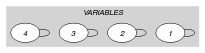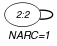## 5.297. open_atmost

Origin
Constraint

$\mathrm{𝚘𝚙𝚎𝚗}_\mathrm{𝚊𝚝𝚖𝚘𝚜𝚝}\left(𝚂,𝙽,\mathrm{𝚅𝙰𝚁𝙸𝙰𝙱𝙻𝙴𝚂},\mathrm{𝚅𝙰𝙻𝚄𝙴}\right)$

Arguments
 $𝚂$ $\mathrm{𝚜𝚟𝚊𝚛}$ $𝙽$ $\mathrm{𝚒𝚗𝚝}$ $\mathrm{𝚅𝙰𝚁𝙸𝙰𝙱𝙻𝙴𝚂}$ $\mathrm{𝚌𝚘𝚕𝚕𝚎𝚌𝚝𝚒𝚘𝚗}\left(\mathrm{𝚟𝚊𝚛}-\mathrm{𝚍𝚟𝚊𝚛}\right)$ $\mathrm{𝚅𝙰𝙻𝚄𝙴}$ $\mathrm{𝚒𝚗𝚝}$
Restrictions
 $𝚂\ge 1$ $𝚂\le |\mathrm{𝚅𝙰𝚁𝙸𝙰𝙱𝙻𝙴𝚂}|$ $𝙽\ge 0$ $\mathrm{𝚛𝚎𝚚𝚞𝚒𝚛𝚎𝚍}$$\left(\mathrm{𝚅𝙰𝚁𝙸𝙰𝙱𝙻𝙴𝚂},\mathrm{𝚟𝚊𝚛}\right)$
Purpose

Let $𝒱$ be the variables of the collection $\mathrm{𝚅𝙰𝚁𝙸𝙰𝙱𝙻𝙴𝚂}$ for which the corresponding position belongs to the set $𝚂$. Positions are numbered from 1. At most $𝙽$ variables of $𝒱$ are assigned value $\mathrm{𝚅𝙰𝙻𝚄𝙴}$.

Example
$\left(\left\{2,3,4\right\},1,〈2,2,4,5〉,2\right)$

The $\mathrm{𝚘𝚙𝚎𝚗}_\mathrm{𝚊𝚝𝚖𝚘𝚜𝚝}$ constraint holds since, within the last three (i.e., $𝚂=\left\{2,3,4\right\}$) values of the collection $〈2,2,4,5〉$, at most $𝙽=1$ value is equal to value $\mathrm{𝚅𝙰𝙻𝚄𝙴}=2$.

Typical
 $𝙽>0$ $𝙽<|\mathrm{𝚅𝙰𝚁𝙸𝙰𝙱𝙻𝙴𝚂}|$ $|\mathrm{𝚅𝙰𝚁𝙸𝙰𝙱𝙻𝙴𝚂}|>1$
Symmetries
• $𝙽$ can be increased.

• An occurrence of a value of $\mathrm{𝚅𝙰𝚁𝙸𝙰𝙱𝙻𝙴𝚂}.\mathrm{𝚟𝚊𝚛}$ can be replaced by any other value that is different from $\mathrm{𝚅𝙰𝙻𝚄𝙴}$.

Arg. properties

Suffix-contractible wrt. $\mathrm{𝚅𝙰𝚁𝙸𝙰𝙱𝙻𝙴𝚂}$.

Keywords
Arc input(s)

$\mathrm{𝚅𝙰𝚁𝙸𝙰𝙱𝙻𝙴𝚂}$

Arc generator
$\mathrm{𝑆𝐸𝐿𝐹}$$↦\mathrm{𝚌𝚘𝚕𝚕𝚎𝚌𝚝𝚒𝚘𝚗}\left(\mathrm{𝚟𝚊𝚛𝚒𝚊𝚋𝚕𝚎𝚜}\right)$

Arc arity
Arc constraint(s)
 $•\mathrm{𝚟𝚊𝚛𝚒𝚊𝚋𝚕𝚎𝚜}.\mathrm{𝚟𝚊𝚛}=\mathrm{𝚅𝙰𝙻𝚄𝙴}$ $•$$\mathrm{𝚒𝚗}_\mathrm{𝚜𝚎𝚝}$$\left(\mathrm{𝚟𝚊𝚛𝚒𝚊𝚋𝚕𝚎𝚜}.\mathrm{𝚔𝚎𝚢},𝚂\right)$
Graph property(ies)
$\mathrm{𝐍𝐀𝐑𝐂}$$\le 𝙽$

Graph model

Since each arc constraint involves only one vertex ($\mathrm{𝚅𝙰𝙻𝚄𝙴}$ is fixed), we employ the $\mathrm{𝑆𝐸𝐿𝐹}$ arc generator in order to produce a graph with a single loop on each vertex. Variables for which the corresponding position does not belong to the set $𝚂$ are removed from the final graph by the second condition of the arc-constraint.

Parts (A) and (B) of Figure 5.297.1 respectively show the initial and final graph associated with the Example slot. Since we use the $\mathrm{𝐍𝐀𝐑𝐂}$ graph property, the loops of the final graph are stressed in bold.

##### Figure 5.297.1. Initial and final graph of the $\mathrm{𝚘𝚙𝚎𝚗}_\mathrm{𝚊𝚝𝚖𝚘𝚜𝚝}$ constraint(a) (b)